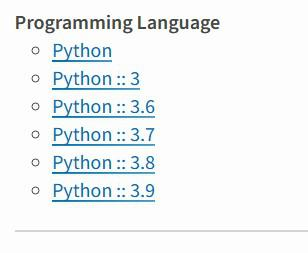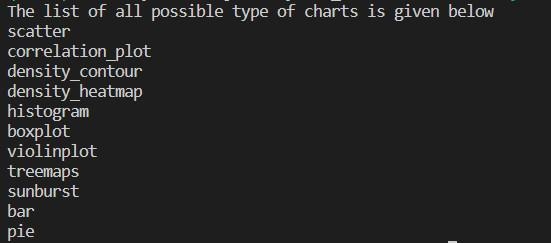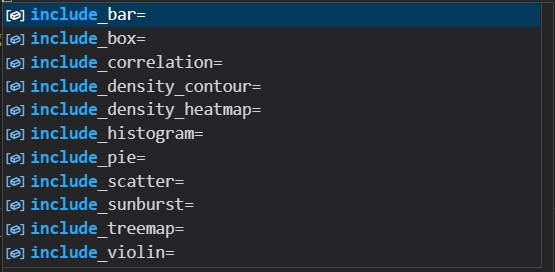Open in App
Not now

# Create Plotly Express charts in less lines of code using Python and Datamallet

• Last Updated : 22 Feb, 2023

In this article, we will see how to create all possible Plotly express charts in very less lines of code using a new Python Module known as datamallet.

### Introduction to the datamallet Library

According to the PyPi website datamallet library is made by Data Scientists to help fellow Data Scientists easily Visualize data in the least amount of line of code possible. It is a collection of different functions of different famous Python libraries, it encapsulates functionalities of Scikit-Learn, Plotly, Pandas, Numpy, and SciPy modules to provide Data Scientists with a one-stop solution for all kinds of Data Visualization and Manipulation.

To install it simply write the following command in the terminal:

`pip install datamallet`

The Datamallet library is only supported by the Python versions mentioned in the following image. It only supports Python 3.9, I have tried to install it in both 3.10 and 3.11 but failed in both, so if the user has Python version <= 3.9 they are good to go.Python versions that support datamallet library

### Importing the Modules

Now we will import pandas This library helps to load the data frame in a 2D array format and has multiple functions to perform analysis tasks in one go. This library helps to load the data frame in a 2D array format and has multiple functions to perform analysis tasks in one go.

## Python3

 `import` `pandas as pd``from` `datamallet.visualization ``import` `AutoPlot`

We will be using the AutoPlot class from the visualization module of datamallet (As it is mentioned earlier that datamallet itself has no special functions, it encapsulates functionalities of different modules).

After importing we will read our dataset using pandas and initialize a variable with the AutoPlot so that we can use that variable further to perform operations. Also, we will use that variable to print out all the possible charts which can be created / available in the visualization module.

## Python3

 `import` `pandas as pd``from` `datamallet.visualization ``import` `AutoPlot` `dataframe ``=` `pd.read_csv(``"dataset.csv"``)` `# Initializing the AutoPlot module``auto ``=` `AutoPlot(df``=``dataframe)` `print``(``"The list of all possible type of charts is given below"``)``for` `i ``in` `auto.chart_type():``    ``print``(i)`

Output:The list of all the possible charts for the dataset at hand

As you can see, we are using a variable dataframe to read and store our csv file and then using the variable auto to initialize AutoPlot by passing our dataframe, df is the parameter that indicates the dataframe which we will visualize. Then using the chart_type() method of AutPlot class to print all the possible list of charts available as a list, as the output of chart_type() is a list we are using a for loop to iterate over it to print all the types in different lines for better understanding.

### Creating all the Plots

The AutoPlot class plots all the plots into an HTML file whose name we have to provide as its parameter while initializing the AutoPlot class. The AutoPlot will create an HTML file named plots inside which it will create all those aforementioned plots. You can download the dataset used from here.

## Python3

 `import` `pandas as pd``from` `datamallet.visualization ``import` `AutoPlot` `dataframe ``=` `pd.read_csv(``"iris.csv"``)` `# Initializing the AutoPlot module``auto ``=` `AutoPlot(df``=``dataframe,filename``=``'plots'``)` `lof ``=` `auto.show()`

Output:

By default, AutoPlot doesn’t plot the following kinds of plots because it would take more time for them to be plotted and a lot more images need to be embedded into that HTML file.Plots that we can draw on the dataset at hand

But we can add this parameter while Initializing AutoPlot to True and those will be added to our HTML file. As shown in the above code we have used the iris dataset and included 11 different types of plots in one go using a single function.

## Python3

 `import` `pandas as pd``from` `datamallet.visualization ``import` `AutoPlot` `dataframe ``=` `pd.read_csv(``"iris.csv"``)` `# Initializing the AutoPlot module``auto ``=` `AutoPlot(df``=``dataframe,filename``=``'plots'``,``    ``include_bar``=``True``,``    ``include_box``=``True``,``    ``include_correlation``=``True``,``    ``include_density_contour``=``True``,``    ``include_density_heatmap``=``True``,``    ``include_histogram``=``True``,``    ``include_pie``=``True``,``    ``include_scatter``=``True``,``    ``include_sunburst``=``True``,``    ``include_treemap``=``True``,``    ``include_violin``=``True``)` `lof ``=` `auto.show()`

Output:

Here I am trying to plot all the possible plots that are available in the module, due to this the HTML file will take a huge time to load, or if the user’s device is not that powerful and the dataset used is huge then it might never open (crash the browser). It is not recommended to try to plot every possible plot at the same time because that will take a huge time and computing power. But for this tutorial’s sake, I am trying to show how the HTML will look if we try to plot every single one.

If we compare this output with the previous output we will see here lots of new plots are being added. If some plots can’t be established due to the dataset (like those plots which require mathematical calculations and must have a certain amount of columns and values or can only be plotted if there are numerical values) then this will not throw any error, only it will not plot those.

My Personal Notes arrow_drop_up